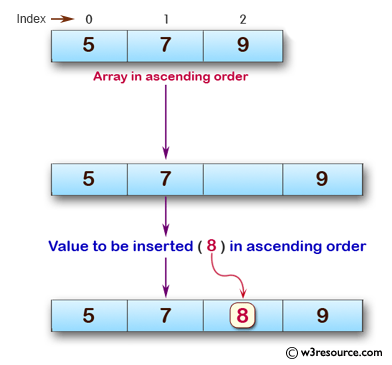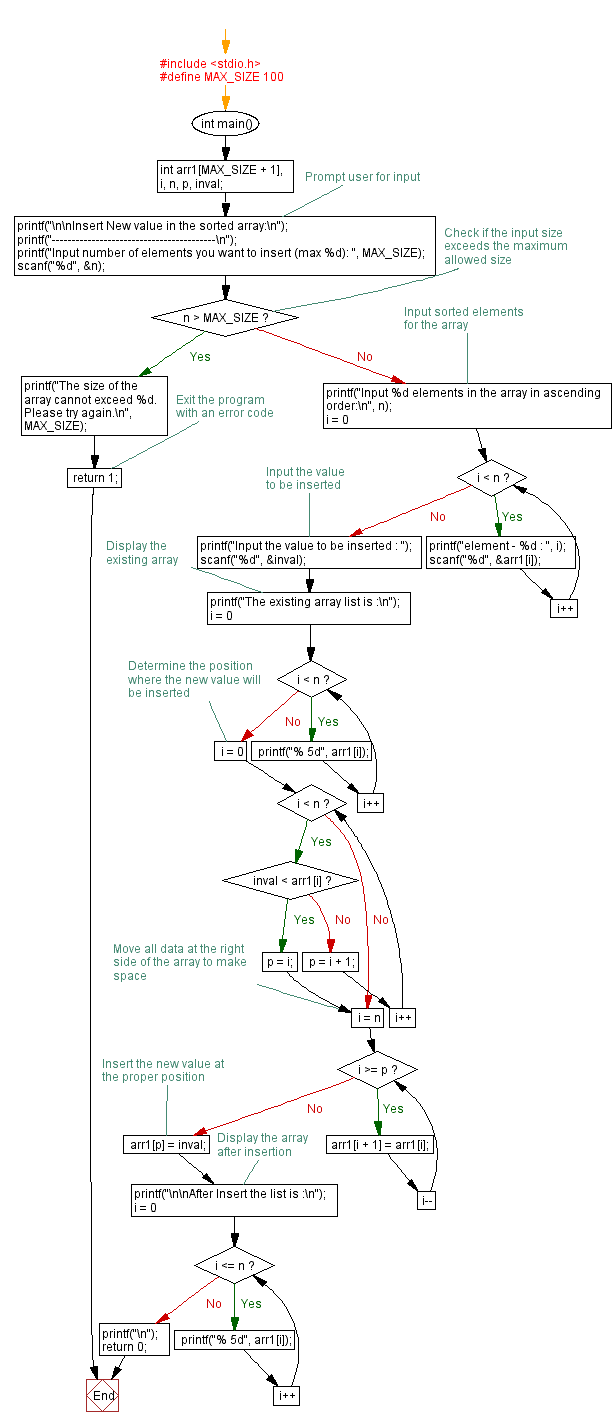﻿ C exercises: Insert New value in the array (sorted list ) - w3resource# C Exercises: Insert New value in the array (sorted list )

## C Array: Exercise-13 with Solution

Write a program in C to insert New value in the array (sorted list ).

Pictorial Presentation:Sample Solution:

C Code:

``````#include <stdio.h>

void main()
{
int arr1,i,n,p,inval;
printf("\n\nInsert New value in the sorted array :\n");
printf("-----------------------------------------\n");

printf("Input the size of array : ");
scanf("%d", &n);
/* Stored values into the array*/
printf("Input %d elements in the array in ascending order:\n",n);
for(i=0;i<n;i++)
{
printf("element - %d : ",i);
scanf("%d",&arr1[i]);
}
printf("Input the value to be inserted : ");
scanf("%d",&inval);
printf("The exist array list is :\n ");
for(i=0;i<n;i++)
printf("% 5d",arr1[i]);
/* Determine the position where the new value will be insert.*/
for(i=0;i<n;i++)
if(inval<arr1[i])
{
p = i;
break;
}
/* move all data at right side of the array */
for(i=n;i>=p;i--)
arr1[i]= arr1[i-1];
/* insert value at the proper position */
arr1[p]=inval;

printf("\n\nAfter Insert the list is :\n ");
for(i=0;i<=n;i++)
printf("% 5d",arr1[i]);
printf("\n");
}
```
```

Sample Output:

```Insert New value in the sorted array :
-----------------------------------------
Input the size of array : 3
Input 3 elements in the array in ascending order:
element - 0 : 5
element - 1 : 7
element - 2 : 9
Input the value to be inserted : 8
The exist array list is :
5    7    9

After Insert the list is :
5    7    8    9
```

Flowchart:C Programming Code Editor:

Improve this sample solution and post your code through Disqus.

What is the difficulty level of this exercise?

﻿